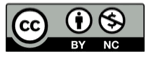# Python 的 函数参数处理机制

2022-01-05## parameter 和 argument 的区别

parameter 出现在函数定义中；argument 出现在函数调用中。

``````# 定义
def f(a):
pass

# 调用
f(1)
``````

## 参数的捕获

• parameters 捕获 arguments 的过程
• argument values 填入 parameter slots 的过程parameter 列表形成一个个的 slot，而 argument 包含若干个 value，通过一系列规定好的逻辑，将 value 捕获到 slot 中去，这就是参数处理机制（parameter handling mechanism），本文的主题围绕这一个捕获过程，系统的梳理一下参数处理机制。

## 通过位置捕获

``````# 定义
def f(a, b):
pass

# 调用
f(1, 2)
``````

• 隐式（implicit）的获取
• 匿名参数``````>>> def f(a,b):
...     pass
...
>>> f(1)
Traceback (most recent call last):
File "<stdin>", line 1, in <module>
TypeError: f() missing 1 required positional argument: 'b'
>>> f(1,2,3)
Traceback (most recent call last):
File "<stdin>", line 1, in <module>
TypeError: f() takes 2 positional arguments but 3 were given
>>>
``````

## 位置参数的困难

``````>>> def f(a,b,c,d,e,f,g):
...     pass
...
>>> f(1,2,3,4,5,6,7)
>>>
``````

``````------>
f(a,b,c,d,e,f,g)
``````

``````------>
f(1,2,3,4,5,6,7)
``````

## 通过名称捕获``````>>> def f(a,b,c,d,e,f,g):
...     pass
...
>>> f(1,2,3,4,5,6,7)
>>> f(g=1,f=2,e=3,d=4,c=5,b=6,a=7)
>>>
``````

## 位置参数和关键字参数的混合``````>>> def f(a,b,c,d,e,f,g):
...     pass
...
>>> f(g=1,f=2,3,4,5,b=6,a=7)
File "<stdin>", line 1
f(g=1,f=2,3,4,5,b=6,a=7)
^
SyntaxError: positional argument follows keyword argument
>>>
``````## 参数默认值``````>>> def f(a,b=1,c):
File "<stdin>", line 1
def f(a,b=1,c):
^
SyntaxError: non-default argument follows default argument
>>>
````````````f(1, 2)
``````## 可变长度位置参数

``````>>> max('a')
'a'
>>> max('a','b','c')
'c'
>>>
````````````>>> def f(*a):
...     print(a)
...
>>> f(1,2,3,4)
(1, 2, 3, 4)
>>>
````````````>>> def f(*a):
...     print(a)
...
>>> b='123'
>>> f(*b)
('1', '2', '3')
>>>
``````

## 可变长度关键字参数

``````>>> def f(**a):
...     print(a)
...
>>> f(b=1, c=2)
{'b': 1, 'c': 2}
>>>
````````````>>> f(**{'b':1,'c':2})
{'b': 1, 'c': 2}
>>>
``````

``````>>> def f(**a):
...     for k,v in a.items():
...         print(k,v,sep='->')
...
>>> f(**{'b d':1,'c':2})
b d->1
c->2
>>>
``````

## 普通位置参数 和 可变长度位置参数的组合

``````>>> def f(name, *inventory):
...     print(name+': '+', '.join(inventory))
...
>>> f('Amy','water','food')
Amy: water, food
>>> f('Bob','car')
Bob: car
>>>
````````````# Python 2.x
>>> def f(*a, b):
File "<stdin>", line 1
def f(*a, b):
^
SyntaxError: invalid syntax
>>>
``````

## 普通位置参数 和 可变长度关键字参数的混合``````>>> def f(name, **inventory):
...     print(name+': '+', '.join([k+': '+v for k,v in inventory.items()]))
...
>>> f('Bob', car='jeep')
Bob: car: jeep
>>>
````````````>>> def f(**inventory, name):
File "<stdin>", line 1
def f(**inventory, name):
^
SyntaxError: invalid syntax
>>>
``````

## 可变长度位置参数 和 可变长度关键字参数的混合

``````>>> def f(*names, **inventory):
...     print(', '.join(names)+': '+', '.join([k+': '+v for k,v in inventory.items()]))
...
>>> f('Amy','Bob', car='jeep')
Amy, Bob: car: jeep
Cindy, David: food: bread, juice: apple
>>>
````````````>>> def f(**inventory, *names):
File "<stdin>", line 1
def f(**inventory, *names):
^
SyntaxError: invalid syntax
>>>
``````

## 精彩评论